NCERT Solutions for Class 8 Maths Chapter 16 Playing with Numbers Ex 16.1 are part of NCERT Solutions for Class 8 Maths. Here we have given NCERT Solutions for Class 8 Maths Chapter 16 Playing with Numbers Ex 16.1.

 Board CBSE Textbook NCERT Class Class 8 Subject Maths Chapter Chapter 16 Chapter Name Playing with Numbers Exercise Ex 16.1 Number of Questions Solved 1 Category NCERT Solutions

## NCERT Solutions for Class 8 Maths Chapter 16 Playing with Numbers Ex 16.1

Question 1.
Find the values of the letters in each of the following and give reasons for the steps involved.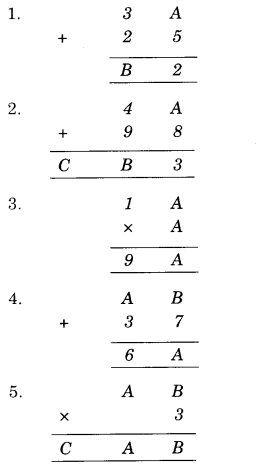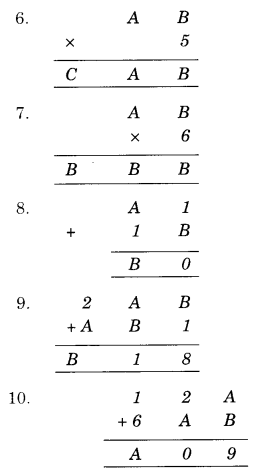Solution.
1.
Here, there are two letters A and B whose values are to be found out.
Let us see the sum in unit’s column. It is A + 5 and we get 2 from this. So,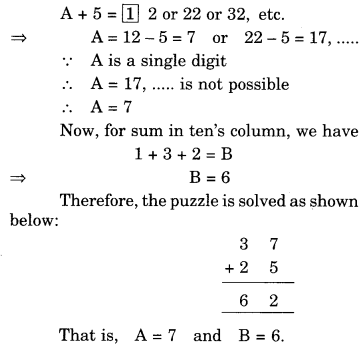2.
Here, there are three letters A, B and C whose values are to be found out.
Let us see the sum in unit’s column. It is A + 8 and we get 3 from this. So A has to be 5That is, A = 5, B = 4 and C = 1.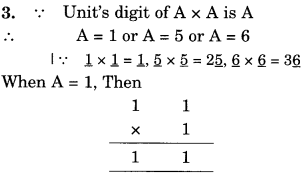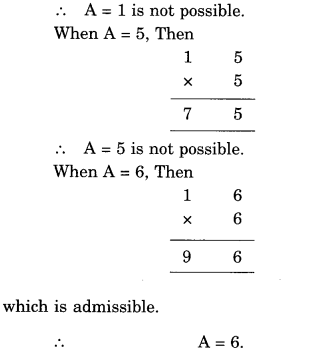4.
Here, there are two letters A and B whose values are to be found out.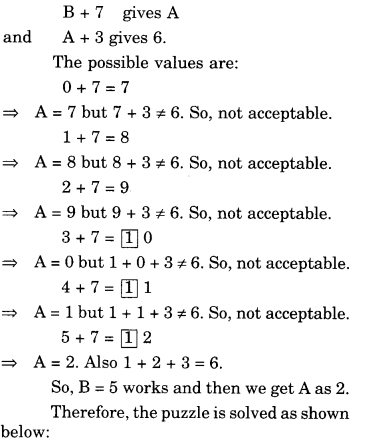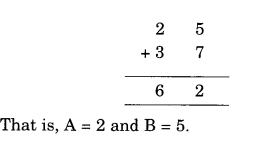5.
Here, there are three letters A, B and C whose values are to be found out.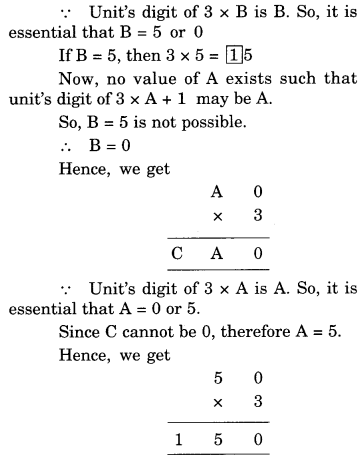∴ A = 5, B = 0 and C = 1

6.
Here, there are three letters A, B and C, whose values are to be found out.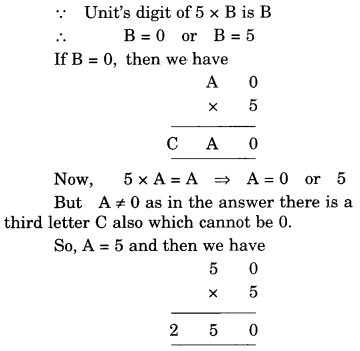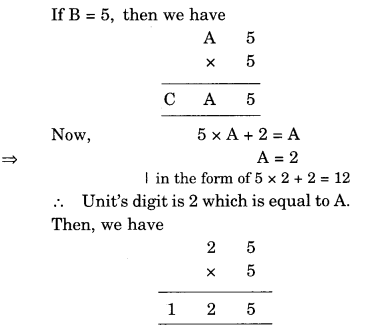7.
Here, there are two letters A and B whose values are to be found out. We have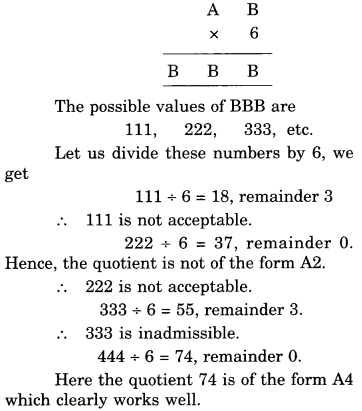8.
Here, there are two letters A and B whose values are to be found out.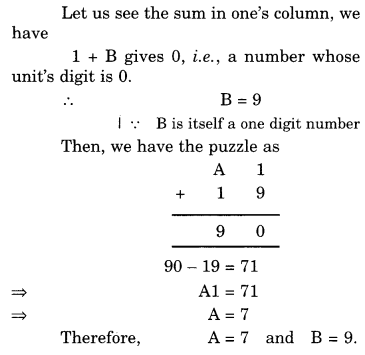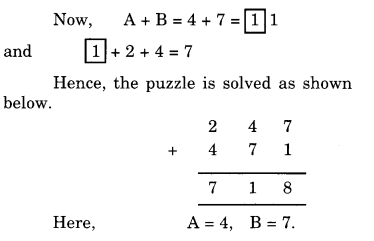10.
We are to find out values of A and BWe hope the NCERT Solutions for Class 8 Maths Chapter 16 Playing with Numbers Ex 16.1 help you. If you have any query regarding NCERT Solutions for Class 8 Maths Chapter 16 Playing with Numbers Ex 16.1, drop a comment below and we will get back to you at the earliest.